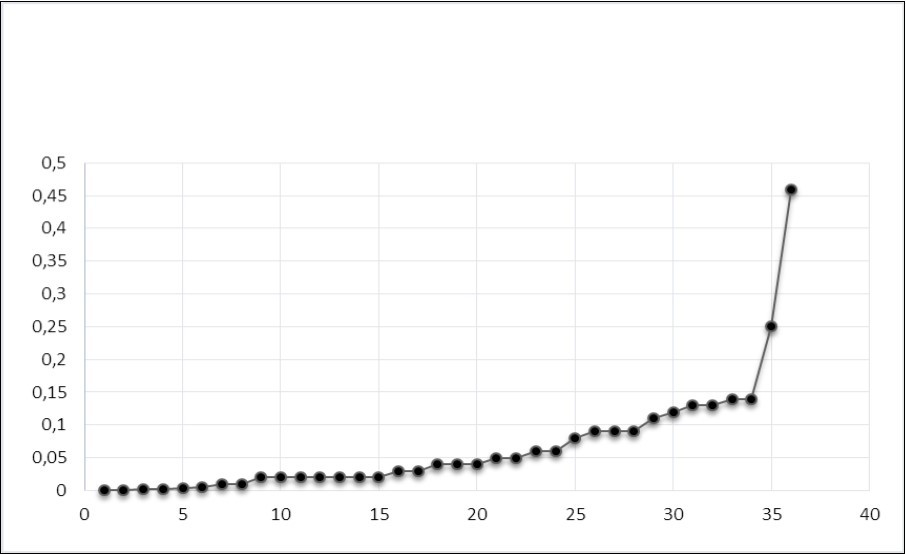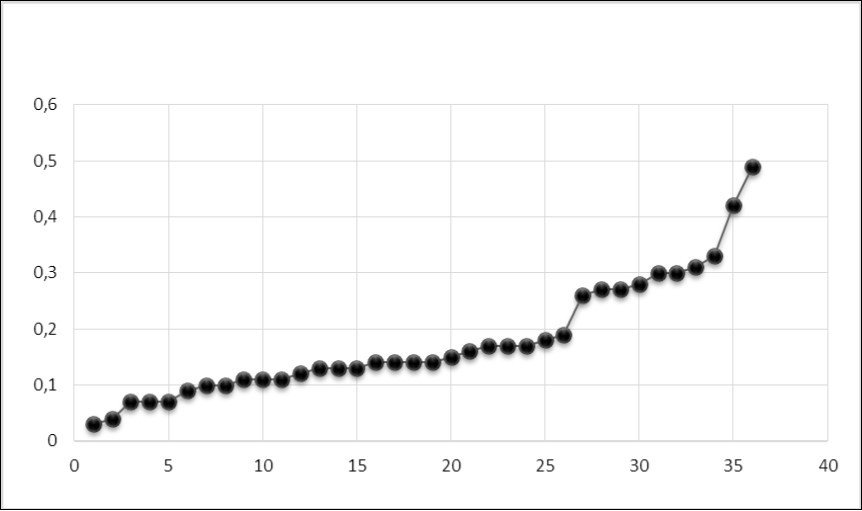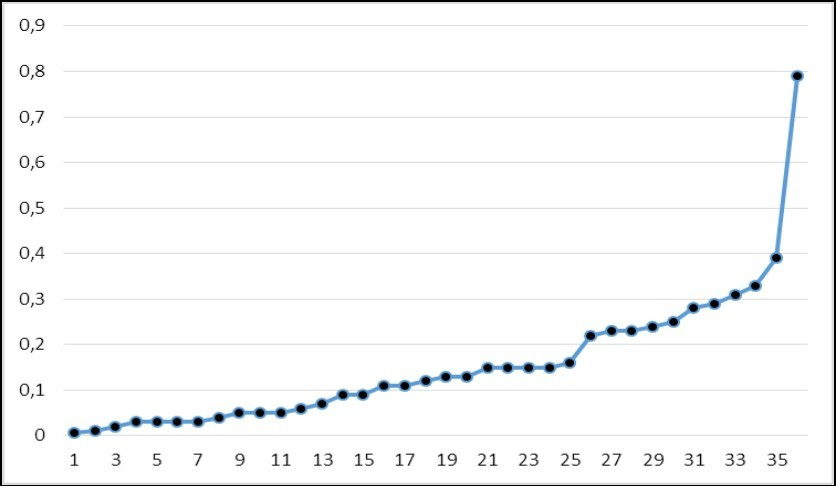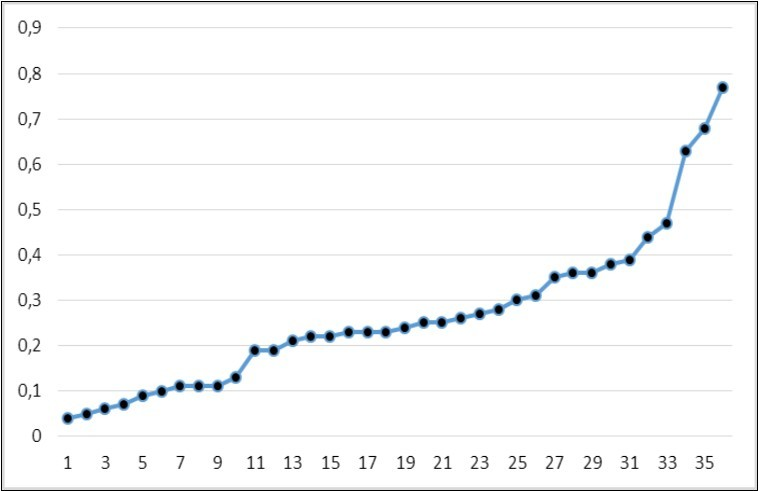### International Journal of Negative Results

Current Issue Volume No: 1 Issue No: 1

Research Article Open Access Available online freely Peer Reviewed Citation

# Membrane Pump Operation Synchronicity (Criticality) in Epidermal Cells: The Possibility of Quantifying the Level of Synchronization

### V.I.Petukhov  1,   Е.V.Dmitriev  2,   L.Kh.Baumane  3,   A.V.Skalny  4,   Yu.N.Lobanova  4,   A.R.Grabeklis  4

2Institute of Numerical Mathematics, Russian Academy of Sciences, Moscow, Russia

3Latvian Institute of Organic Synthesis, Riga, Latvia

4ANO Centre for Biotic Medicine, Moscow, Russia

## Abstract

The authors analyze the results of atomic emission spectrometry of hair samples for Al, Cd, Fe, Cr, Cu, Li, Pb, V, and Zn in 952 healthy subjects and 952 liquidators of the Chernobyl nuclear power plant accident. Using correlation analysis (Pearson) of the obtained data, the authors have found pair correlations between metal concentration values. According to the authors, criticality or synchronization (as a particular case of the critical state of the system) can be inherent in all ATP-dependent membrane pumps (P-type) controlling metal-ligand homeostasis in epidermal cells. A quantitative criterion (synchronization index) is proposed to measure the level of criticality (synchronization) in the functioning of membrane ATPases.

##### Author Contributions
Received 16 Jul 2018; Accepted 29 Aug 2018; Published 01 Sep 2018;

Academic Editor: Anoja Priyadarshani Attanayake, Senior Lecturer in Biochemistry, Department of Biochemistry, Faculty of Medicine, University of Ruhuna, Sri Lanka.

Checked for plagiarism: Yes

Review by: Single-blindThis is an open-access article distributed under the terms of the Creative Commons Attribution License, which permits unrestricted use, distribution, and reproduction in any medium, provided the original author and source are credited.

Competing interests

The authors have declared that no competing interests exist.

Citation:

V.I.Petukhov , Е.V.Dmitriev , L.Kh.Baumane , A.V.Skalny , Yu.N.Lobanova et al. (2018) Membrane Pump Operation Synchronicity (Criticality) in Epidermal Cells: The Possibility of Quantifying the Level of Synchronization. International Journal of Negative Results - 1(1):12-19. https://doi.org/10.14302/issn.2641-9181.ijnr-18-2226

## Introduction

For over a decade, the authors have been using hair samples as a bio-substrate for non-invasive studies of metal-ligand homeostasis (MLH). Our experience allows us to make the following preliminary conclusions:

1) Quantitative assessment of the metal content in the given substrate (hair) is not representative of the whole body essential metal availability of (save for extreme forms of metal deficiency or overabundance) and, therefore, cannot serve as a reliable diagnostic criterion for latent MLH disturbances;

2) Having found evidence of critical (synchronous) nature of the operation of K/Na-ATPase 1 controlling K/Na-homeostasis in epidermis as well as other ATPases from the P-type family that control transmembrane traffic of Cu, Zn, Fe 2, we can classify MLH in these cells as self-organized criticality phenomena (SC phenomena);

3) Regulatory control of MLH in epidermal cells occurs most likely (and mostly) by the natural law of self-organized criticality 3 whose relevance to cellular energy exchange seems highly probable since a living cell is an open dynamic system;

4) Since criticality or synchronization (as a particular case of the critical state of a system) can be inherent in all ATP-dependent membrane pumps (P-type) controlling the MLH of epidermis, it is of interest to quantify the synchronization (criticality) of membrane pump operation.

The present article is an attempt to obtain such an estimate. The proposed approach is based on the fundamental principles of the self-organized criticality theory and the modern concept of a three-component biosystem existing in the cell and consisting of nitric oxide (NO), free thiols and non-heme iron 4. Within the framework of this system, S-nitrosothiols (RS-NO) and dinitrosyl iron complexes with thiol-containing ligands (DNICs) are continuously synthesized, both in mononuclear [(RS)2Fe(NO)2] – M-DNICs, and binuclear form [(RS)2Fe2(NO)4] – B-DNICs. In the self-oscillation mode (by the Belousov-Zhabotinsky reaction), there is constant inter-transformation of RS-NO and DNICs whereby the most reactogenic forms of NO are formed: nitrosonium (NO+) ions and nitroxyl (NO or HNO) which have a longer lifetime as compared to nitrogen monoxide. As a result, conditions are created for stable (long-term) functioning of the short-lived NO molecule as a universal manager 4.

Nitric oxide (NO) or, more precisely, its active derivatives (nitrosonium and nitroxyl ions) act as ATPase activators, due to S-nitrosylation of SH-groups of cysteine in the protein molecules of these pumps. Reactive oxygen species (ROS) also have the ability to redox-modify amino acid residues to form disulfide bonds.

Activated ATPase molecules on the cell membrane form a ‘system of weakly interacting oscillators’ (in terms of the self-organized criticality theory) which (upon reaching the threshold density) allows for the possibility of their spontaneous self-organization - transition to a critical state or synchronization.

The aforementioned events are evidenced by some facts as shown below.

## Material and Methods

At the Biotic Medicine Centre (Moscow), mineral composition of hair was analysed by the atomic emission spectrometry method in 952 healthy subjects (238 males and 714 females aged 2 to 86) and 952 liquidators of the consequences of the Chernobyl NPP accident – Moscow residents (213 females and 739 males aged 37 to 82).

Hair samples for spectrometric studies were obtained upon subjects’ voluntary informed consent to the tests. A bundle of hair 2 cm long and 0.5 cm thick was cut off in the occipital region close to the scalp skin. The procedure for preparing a hair sample for spectrometric analysis was the same as in our previous work 2.

Hair metal content was assessed by inductively coupled plasma mass spectrometry (ICP-MS) with NexION 300D (Perkin Elmer Inc., Shelton, CT, USA) using Dynamic Reaction Cell technology removing the majority of interferences with little or no loss of analyte sensitivity and equipped with ESI SC-2 DX4 autosampler (Elemental Scientific INC., Omaha, NE, USA).

We have analyzed the results of atomic emission spectrometry of hair samples for Al, Cd, Fe, Cr, Cu, Li, Pb, V and Zn. A correlation analysis (Pearson) of the obtained data was conducted with finding of pair correlations between the concentration values of metals ("each with each") in 36 pairs: Al-Cd, Al-Fe, Al-Cr, Al-Cu, Al-Li, Al-Pb, Al-V, Al-Zn, Cd-Fe, Cd-Cr, Cd-Cu, Cd-Li, Cd-Pb, Cd-V, Cd-Zn, Fe-Cr, Fe-Cu, Fe-Li, Fe-Pb, Fe-V, Fe-Zn, Cr-Cu, Cr-Li, Cr-Pb, Cr-V, Cr-Zn, Cu-Li, Cu-Pb, Cu-V, Cu-Zn, Li-Pb, Li-V, Li-Zn, Pb-V, Pb-Zn, V-Zn.

The terms used herein require explanation. We use the terms ‘hair’ and ‘epidermal cells (epidermocytes)’ as synonyms since it is known that hair is derivative of epidermis. However, the core of the human hair consists of small, not completely keratinized epithelial cells, which in the volume usually do not exceed 1/3 of the hair thickness. This allows you to study the events occurring in the MLH epidermis, practically on-line.

The trapezium method was used to measure the area on the graph under the curve of pair correlation coefficients (r). The Matlab software tool was used for statistical data processing.

## Results and Discussion

In our previous studies of MLH in a group of Chernobyl liquidators 5, 6, we discovered that the content of such metals as Al, Cd, Fe, Cr, PB, V were higher, while the content of Cu and Zn were lower than the norm (Table 1).

Table 1. The content of metals in epidermal cells of the Chernobyl liquidators and healthy subjects 1
 Zn Metals Healthy subjects(n = 947), μg/g Chernobyl liquidators (n = 954), μg/g Healthy subjects (n=947), μg/g Group Ir K-Zn = -0.01(n = 253) Group IIr K-Zn = -0.43(n = 171) Al 8.1<8.8<9.5 19.3<20.1<20.9* 4.3<5.2<6.2 10.8<12.3<14.2* Cd 0.04<0.05<0.06 0.2<0.25<0.3* 0.027<0.03<0.04 0.05<0.06<0.08* Fe 19.3<21.07<23.1 22.4<23.7<25.07** 15.0<17.8<21.5 20.0<22.5<24.9** Cr 0.48<0.51<0.54 0.85<0.9<0.92* 0.35<0.39<0.42 0.62<0.72<0.83* Cu 19.06<20.7<22.3 10.6<10.99<11.4* 18.6<21<23.5 14.3<16<18.2* Li 0.03<0.04<0.05 0.05<0.06<0.062* 0.02<0.023<0.03 0.046<0.05<0.06* Pb 1.04<1.1<1.27 1.5<1.8<2.2* 0.68<0.95<1.3 1.1<1.4<1.6** V(female) 0.058<0.06<0.07 0.10<0.12<0.13* 0.05<0,06<0.07 0.08<0.09<0.11* V(male) 0.08<0.09<0.1 0.11<0.116<0.12* 0.05<0.06<0.07 0.09<0.11<0.13* 181.5<185.2<189.3 162.5<165.8<169* 196.0<204.3<213.5 143.2<150.7<158.3*

* Note: Since it is impossible to confirm the normal distribution hypothesis, one must abandon the standard estimation methods and choose the bootstrap-method 11. Mean values are shown in bold, plain script shows confidence interval (bootstrap-method); statistical significance of differences is marked with asterisks: p< 0.001;
** p<0.05.

It was assumed that these shifts in MLH were associated with increased production of reactive oxygen (ROS) and nitrogen (RNS) species, i.e. oxidative/nitrosative stress in the Chernobyl liquidators.

To verify the above assumption, we performed a quantitative assessment of the nitric oxide level in the epidermis (hair) by electron paramagnetic resonance (EPR) using diethylthiocarbamate (DETC) as a trap for NO in vitro. According to the EPR analysis, the NO level in the epidermis of the Chernobyl liquidators was significantly higher than normal 7.

At the same time, it was found that the absolute majority of the accident liquidators (88%) showed a negative and significant K-Zn correlation (in 205 persons r K-Zn= -0.62; p<0.05; in 634 persons r K-Zn=-0.41; p<0.05). In 12% of the Chernobyl liquidators (115 persons), it was not detected (r = -0.03) 5. The distribution of the groups studied with different values of rK-Zn was carried out according to the technique developed by us 8.

The K-Zn correlation was absent (r = -0.01) in 253 healthy subjects (26,7%), feebly marked (r K-Zn = -0,22; p<0,05) in 523 persons (55,2%) and obviously marked (r K-Zn= -0.43; p<0.05) in 171 persons (18.1%) only.

The analysis of metal homeostasis in epidermal cells of healthy subjects at the extreme values of rK-Zn (-0.01 and -0.43) has shown that shifts in MLH of healthy persons with rK-Zn = -0.43 were similar to those of the total group of the Chernobyl liquidators (n=954) compared with the control group (n=947) 1. One can draw a conclusion, (which seems obvious although requiring verification) about the presence of oxidative/nitrosative stress of non-radiation nature in healthy subjects with rK-Zn = -0.43 (Table 1).

Similar changes in concentration values of metals dependent on the value of |r|K-Zn were also found in the Chernobyl liquidators 1.

It can be assumed that the negative correlation ratio K-Zn in the setting of oxidative/nitrosative stress really reflects the synchronous operation of the superfamily of membrane ATPases (which is indirectly suggested by the spectrometry results), the revealed ‘sensitivity’ of metals to increased production of ROS/RNS, which, alongside with the growth of |r|K-Zn, is accompanied by notable shifts in intracellular level of metals, lead us to the unequivocal conclusion that normal (unfailing) operation of membrane pumps must take place in the synchronous mode, that is, in the self-organized criticality mode.

In our recent studies, we were able to detect the signs of a critical period in MLH (due to the presence of a power-law connection between the concentration values) for such metals as Na, K, Ca 1, and also Fe, Cu and Zn 2. The fractal character of these metal concentration distribution (not dependent on the size of the system) can also indicate the belonging of the homeostasis of these metals to SC phenomena.

According to the results of the correlation analysis (coefficients r of linear paired correlations without the sign), the graphs were constructed where r-values form a curve (Figure 1, Figure 2, Figure 3, and Figure 4). The area under this curve, calculated using the trapezium method, was used as the quantitative criterion for synchronization (criticality) - the synchronization index (SI).

As shown in the figures - in groups with n = 952 (Figure 1- Figure 2), the area under the curve of |r| or the synchronization index (SI) for the Chernobyl liquidators was almost twice that of healthy subjects (9.17 vs 5.18).

Figure 1.The coefficients |r| for paired correlations (Pearson) among the concentration values of metals (Al, Cd, Fe, Cr, Cu, Li, Pb, V, Zn) in healthy subjects (n = 952). The ordinate represents the values of |r|; on the abscissa - the pair number with increasing |r|. The area under the curve is the synchronization index (SI = 2.17).Figure 2.The coefficients |r| pair correlations (Pearson) among the concentration values of metals (Al, Cd, Fe, Cr, Cu, Li, Pb, V, Zn) in the Chernobyl liquidators (n = 952). The ordinate represents the values of |r|; on the abscissa - the pair number with increasing |r|. The area under the curve is the synchronization index (SI = 6.13).In healthy subjects with n = 76 and different values of rK-Zn (Figure 3- Figure 4), the SI value in healthy individuals with rK-Zn = -0.43 is also almost 2 times higher than in the group with r K-Zn = -0.01. The total sum of values of the coefficient |r| (without the sign) depended on the sample size: it was larger with smaller sample sizes. Apparently, the explanation of this fact should be sought in the stochastic character of pair correlations (these can be more easily detected with a decrease in the sample size).

It is significant that the ‘increment’ of SI was independent of the sample size - it was almost the same for groups with n = 952 (Figure 1 and Figure 2) and with n = 76 (Figure 3- Figure 4): 3.96 and 3.99 resp. At the same time, the increase of SI among the Chernobyl liquidators in comparison with the norm and in healthy subjects with negative K-Zn correlation (r K-Zn = -0.43) was accompanied by coincident shifts in the magnitude and direction of the MLH: an increase in the level of Al, Cd, Fe, Cr, Li, Pb, V, and a decrease in Cu and Zn in epidermal cells (Table 2).

Figure 3.The coefficients |r| (Pearson) among the concentration values of metals (Al, Cd, Fe, Cr, Cu, Li, Pb, V, Zn) in healthy subjects in the group with r K-Zn = -0.01 (n = 76). The ordinate represents the values of |r|; on the abscissa - the pair number with increasing |r|. The area under the curve is the synchronization index (SI = 5.18).Figure 4.The coefficients |r| (Pearson) among the concentration values of metals (Al, Cd, Fe, Cr, Cu, Li, Pb, V, Zn) in healthy individuals in the group with r K-Zn = -0.43 (n = 76). The ordinate represents the values of |r|; on the abscissa - the pair number with increasing |r|. The area under the curve is the synchronization index (SI = 9.17).Table 2. The content of metals and SI in epidermal cells of the Chernobyl liquidators and healthy subjects with different level of r K-Zn
 Metals Median (Me) (μg/g) Median (Me) in healthy subjects (μg/g) with different rK-Zn Chernobyl liquidators (n=952) Healthy subjects (n=952) r K-Zn= - 0,43(n=76) r K-Zn= -0.01(n=76) Al ↑ 17.1 6.05 ↑ 10.3 2.58 Cd ↑ 0.16 0.01 ↑ 0.03 0.01 Fe ↑ 18.4 17,5 ↑ 18.4 9.6 Cr ↑ 0.77 0.35 ↑ 0.62 0.28 Cu ↓ 9.9 15.8 ↓ 12.1 14.2 Li ↑ 0.03 0.02 ↑ 0.05 0.01 Pb ↑ 0.81 0.51 ↑ 1.15 0.22 V ↑ 0.08 0.05 ↑ 0.08 0.03 Zn ↓ 167.9 210.5 ↓ 137.1 190.6 SI(synchronization index) 6.13 2.17 9.17 5.18

Note: Arrows indicate changes in intracellular metal content
( - an increase)
( - a decrease).

In our opinion, these changes in MLH should be explained by the synchronous functioning of ATPase (P-type), which are responsible for the transmembrane traffic of these metals 9, 10. At the same time, the increased production of nitric oxide in the liquidators of the accident in the same substrate (hair), which we previously showed by increasing the NO signal on the EPR spectrogram 7, led to an increase in the synchronization level (SI). Similar dynamics was observed in the healthy group (n = 76) with different values of r K-Zn (see Table 2). As already mentioned, we do not exclude the presence of oxidative/nitrosative stress) of non-radiation origin in healthy subjects with r K-Zn = -0.43.

The sequence of events occurring in the epidermis, which lead to a synchronous (critical) mode of operation of membrane ATPases, may be as follows:

Generation of NO+ and HNO by self-oscillating RS-NO↔DNICs systems in a larger volume (Chernobyl liquidators and healthy subjects with rK-Zn = -0.43) induces to reach the threshold density of activated ATPases of cell membrane. In a system of weakly interacting oscillators, which is the ATPase system, this inevitably leads to self-organization of the system or to a critical (synchronous) mode of operation. One of the criteria for the critical state of the system is the value of the so-called branching parameter σ, equal to the average number of the nearest neighbors to which each of the oscillators transmits an exciting pulse. In the critical state, σ is equal to 1 (σ=1). Along with the critical, subcritical (σ <1) and supercritical (σ > 1) states (or self-organization regimes) are distinguished.

In our opinion, the following questions would be of utmost interest in further research into this problem.

Is the role of the RS-NO↔DNICs system with respect to membrane pumps restricted to uninterrupted production of ATPase activators (NO+ and HNO) in the cell, and how does the oscillatory character of the functioning of the RS-NO↔DNICs system affect the operation of membrane pumps? There are no explicit answers to these questions yet, which encourages further research in the given field.

## Conclusion

In conclusion, it should be noted that the main points of the self-organized criticality theory, as our experience shows, help explain some complex and understudied processes in the MLH of epidermal cells.